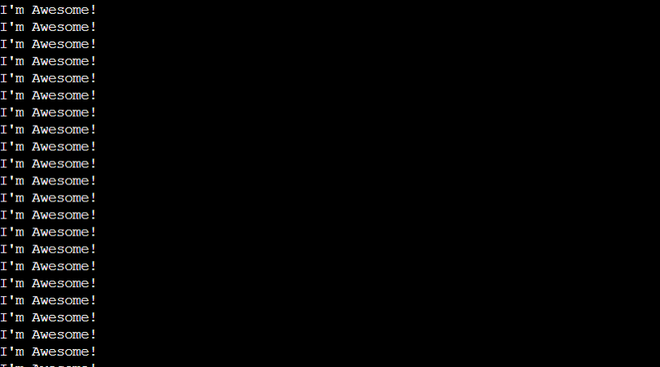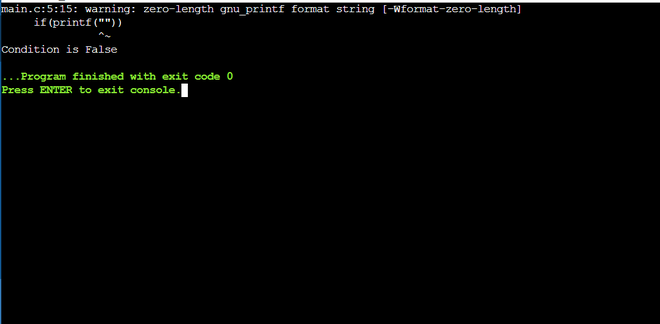GFG App
Open AppBrowser
Continue

# What will happen if a print() statement is written inside a if() such as if(print())

Pre-requisite: if-else

This article focuses on discussing what happens when the print statement is used inside the if-else conditional statement.

For example: Consider the below code and predict the output.

## C

 `// C program`   `#include `   `// Driver code` `int` `main()` `{` `    ``// if statement` `    ``if` `(``printf``(``"I'm Awesome!\n"``))`   `        ``// calling main() function` `        ``main();`   `    ``// else-if statement` `    ``else` `if` `(``printf``(``"I'm Not Awesome\n"``))`   `        ``// calling main() function` `        ``main();` `}`

## C++

 `// C++ program`   `#include ` `using` `namespace` `std;`   `// Driver code` `int` `main()` `{` `    ``// if statement` `    ``if` `(``printf``(``"I'm Awesome!\n"``))`   `        ``// calling main() function` `        ``main();`   `    ``// else-if statement` `    ``else` `if` `(``printf``(``"I'm Not Awesome\n"``))`   `        ``// calling main() function` `        ``main();` `    ``return` `0;` `}`

## Java

 `// Java program to implement the above approach` `class` `GFG {`   `    ``public` `static` `void` `main(String[] args) {`   `        ``// if statement` `        ``if` `(System.out.printf(``"I'm Awesome!\n"``) != ``null``)`   `            ``// calling main() function` `            ``main(``null``);`   `        ``// else-if statement` `        ``else` `if` `(System.out.printf(``"I'm Not Awesome\n"``) != ``null``)`   `            ``// calling main() function` `            ``main(``null``);` `    ``}` `}` `// This code contributed by Princi Singh`

## Python3

 `# Python program` `# Driver code` `# if statement` `if``(``print``(``"I'm Awesome!"``)):` `    `  `   ``# calling main() function` `   ``def` `main():` `       `  `# else-if statement` `elif``(``print``(``"I'm Not Awesome"``)):` `            `  `   ``# calling main() function` `   ``def` `main():`   `# This code is contributed by shivanisinghss2110`

## C#

 `// C# program to implement the above approach` `using` `System;` `class` `GFG {`   `    ``public` `static` `void` `Main(String[] args) {`   `        ``// if statement` `        ``if` `(Console.WriteLine(``"I'm Awesome!\n"``))`   `            ``// calling Main() function` `            ``Main(``null``);`   `        ``// else-if statement` `        ``else` `if` `(Console.Write(``"I'm Not Awesome\n"``))`   `            ``// calling Main() function` `            ``Main(``null``);` `    ``}` `}`   `// This code is contributed by shivanisinghss2110`

## Javascript

 ``

Guessing the Expected Output: Most general guess for the output of this program is some sort of ERROR.

Actual Output: But its actual output is a bit strange and not the expected one.Explanation: Let’s discuss the reason.

• As if condition is being satisfied every time and due to recursive call of the main() function, this function call will ideally go on infinitely but in the real scenario, it’ll stop once the allowed function call stack will be filled.
• So, first of all, let’s understand why the print function inside if condition is not giving error and how it’s working internally.
• This behavior is based on a simple fact that print function like printf in C/C++ etc returns an integer value equal to the number of characters it has printed successfully.

Let’s understand this by a program below-

## C

 `// C program to implement` `// the above approach` `#include `   `// Driver code` `int` `main()` `{` `    ``// Nested printf function` `    ``printf``(``"%d"``, ``printf``(``"GFG!\n"``));` `    ``return` `0;` `}`

## C++

 `// C++ program to implement` `// the above approach` `#include ` `using` `namespace` `std;`   `// Driver code` `int` `main()` `{` `    ``// nested printf function` `    ``printf``(``"%d"``, ``printf``(``"GFG!\n"``));` `    ``return` `0;` `}`

## Java

 `// Java program to implement` `// the above approach`   `import` `java.util.*;`   `class` `GFG{`   `// Driver code` `public` `static` `void` `main(String[] args)` `{` `    ``// nested printf function` `    ``System.out.printf(``"%s"``, System.out.printf(``"GFG!\n"``));` `}` `}`   `// This code is contributed by 29AjayKumar`

## Python3

 `# Python program to implement` `# the above approach`   `# Nested printf function` `print``(``print``(``"GFG!\n"``))` `    `  `# This code is contributed by shivanisinghss2110`

## C#

 `// C# program to implement` `// the above approach`   `using` `System;`   `class` `GFG{`   `// Driver code` `public` `static` `void` `Main(String[] args)` `{` `    ``// nested printf function` `    ``Console.Write(``"GFG!\n"``);` `}` `}` ` `    `// This code is contributed by shivanisinghss2110`

## Javascript

 ``

Output

```GFG!
5```

Explanation: When the printf statement is encountered it goes to the outer printf function and tries to print its value and when the program tries to print its value then it encounters another printf and it goes to inner printf (like a recursive call) to execute it and then it executes it and that inner printf function returns 5 because that function has successfully printed 5 characters (‘G’, ‘F’, ‘G’, ‘!’, and ‘\n’) and hence return an integer value equal to 5 in this case.

Will print() used inside if always return true?

From the above example, it can be easily understood how printf functions are working inside if statements and how the condition is being satisfied. The condition will always satisfy until and unless an empty string is printed like as shown in the below code-

The only idea behind this example is to demonstrate if the printf function returns “0” only then the if condition would turn out to be false. In all other cases, it’ll be true whether the length of the string is 1 or 9999.

## C

 `// C program to implement` `// the above approach` `#include `   `// Driver code` `int` `main()` `{` `    ``// printf function inside if statement` `    ``if` `(``printf``(``""``))`   `        ``// printf function` `        ``printf``(``"Condition is True"``);`   `    ``// else statement` `    ``else`   `        ``// printf function` `        ``printf``(``"Condition is False"``);` `    ``return` `0;` `}`

## C++

 `// C++ program to implement` `// the above approach` `#include ` `using` `namespace` `std;`   `// Driver code` `int` `main()` `{` `    ``// printf function inside if statement` `    ``if` `(``printf``(``""``))`   `        ``// printf function` `        ``printf``(``"Condition is True"``);`   `    ``// else statement` `    ``else`   `        ``// printf function` `        ``printf``(``"Condition is False"``);` `    ``return` `0;` `}`

## Java

 `// Java program to implement` `// the above approach` `import` `java.io.*;`   `class` `GFG ` `{`   `// Driver code` `public` `static` `void` `main (String[] args) ` `{` `  `  `    ``// printf function inside if statement` `    ``if` `(System.out.print(``""``))`   `        ``// printf function` `        ``System.out.print(``"Condition is True"``);`   `    ``// else statement` `    ``else`   `        ``// printf function` `        ``System.out.print(``"Condition is False"``);` `}` `}`   `// This code is contributed by shivanisinghss2110.`

## Python3

 `# Python program to implement` `# the above approach`   `    ``# print function inside if statement` `    ``if` `(``print``("")):`   `        ``# print function` `        ``print``(``"Condition is True"``)`   `    ``# else statement` `    ``else``:`   `        ``# print function` `        ``print``(``"Condition is False"``)`   `# This code is contributed by shivanisinghss2110.`

## C#

 `// C# program to implement` `// the above approach` `using` `System;`   `class` `GFG ` `{`   `// Driver code` `public` `static` `void` `Main (String[] args) ` `{` `  `  `    ``// printf function inside if statement` `    ``if` `(Console.Write(``""``))`   `        ``// printf function` `        ``Console.Write(``"Condition is True"``);`   `    ``// else statement` `    ``else`   `        ``// printf function` `        ``Console.Write(``"Condition is False"``);` `}` `}`   `// This code is contributed by shivanisinghss2110`

## Javascript

 ``My Personal Notes arrow_drop_up